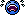# Finding the wave speeds

## Homework Statement

Two steel guitar strings have the same length. String A has a diameter of 0.50 mm and is under 410.0 N of tension. String B has a diameter of 1.0 mm and is under a tension of 820.0 N. Find the ration of the wave speeds, VA / VB
in these two strings.

V= ƒ λ
FT = FG

## The Attempt at a Solution

I'm lost on where to start.....Homework Helper
Gold Member

## Homework Statement

Two steel guitar strings have the same length. String A has a diameter of 0.50 mm and is under 410.0 N of tension. String B has a diameter of 1.0 mm and is under a tension of 820.0 N. Find the ration of the wave speeds, VA / VB
in these two strings.

V= ƒ λ
FT = FG

## The Attempt at a Solution

I'm lost on where to start.....Amongst your standard equations, you should have one that relates wave speed in a string/wire to tension and mass per unit length.

utkarsh009

## Homework Statement

Two steel guitar strings have the same length. String A has a diameter of 0.50 mm and is under 410.0 N of tension. String B has a diameter of 1.0 mm and is under a tension of 820.0 N. Find the ration of the wave speeds, VA / VB
in these two strings.

V= ƒ λ
FT = FG

## The Attempt at a Solution

I'm lost on where to start.....v=√(T/¢)
T= tension in wire
¢= mass per unit length